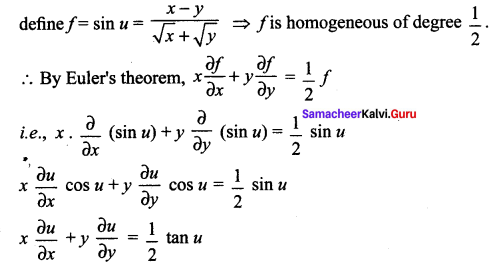You can Download Samacheer Kalvi 12th Maths Book Solutions Guide Pdf, Tamilnadu State Board help you to revise the complete Syllabus and score more marks in your examinations.

## Tamilnadu Samacheer Kalvi 12th Maths Solutions Chapter 8 Differentials and Partial Derivatives Ex 8.7

Question 1.
In each of the following cases, determine whether the following function is homogeneous or not. If it is so, find the degree.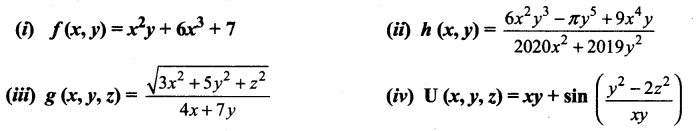Solution: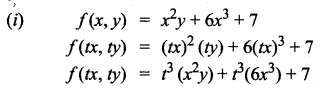It is not a homogeneous function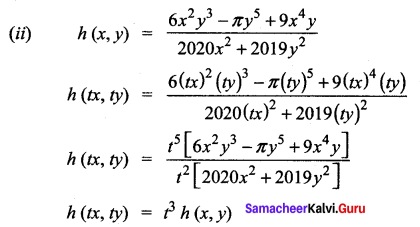∴ It is a homogeneous function with degree 3.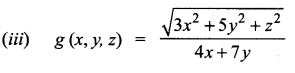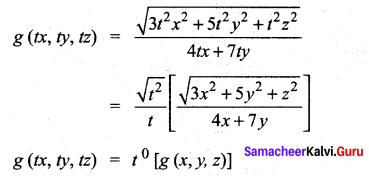∴ It is homogeneous function of degree 0.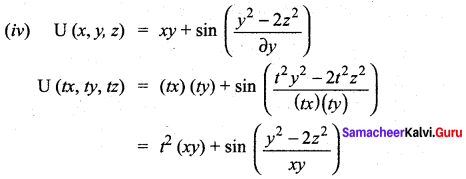∴ It is not a homogeneous functionQuestion 2.
Prove that f(x, y) = x3 – 2x2 y + 3xy2 + y3 is homogeneous; what is the degree? Verify Euler’s Theorem for f.
Solution:
f (x, y) = x3 – 2x2y + 3xy2 + y3
f(tx, ty) = t3x3 – 2(t2x2)(ty) + 3(tx)(t2y2) + t3y3
= t3 [x3 – 2x2y + 3xy2 + y3]
f(tx, ty) = t3 f(x, y)
‘f’ is a homogeneous function of degree 3. By Euler’s theorem, we have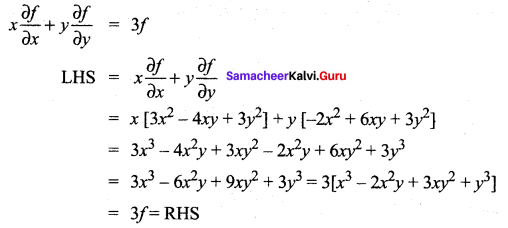∴ Euler’s Theorem verified

Question 3.
Prove that g(x, y) = x log ($$\frac{y}{x}$$) is homogeneous; what is the degree? Verify Euler’s Theorem for g.
Solution: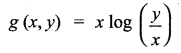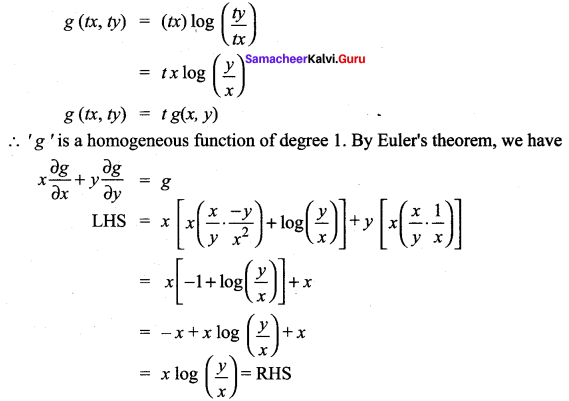∴ Euler’s Theorem verifiedQuestion 4.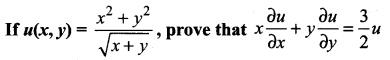Solution: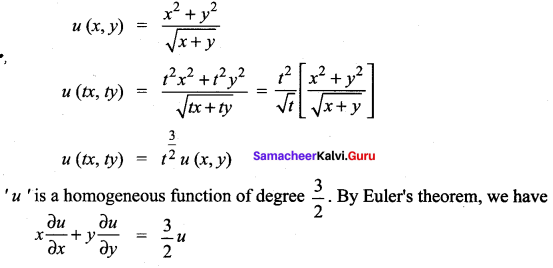Question 5.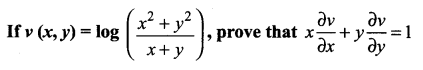Solution: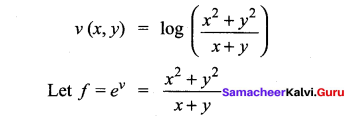∴ ‘f’ is a homogeneous function of degree 1. By Euler’s theorem, we have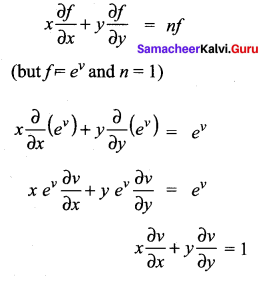Question 6.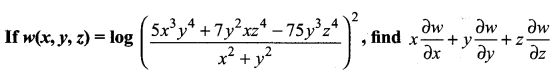Solution: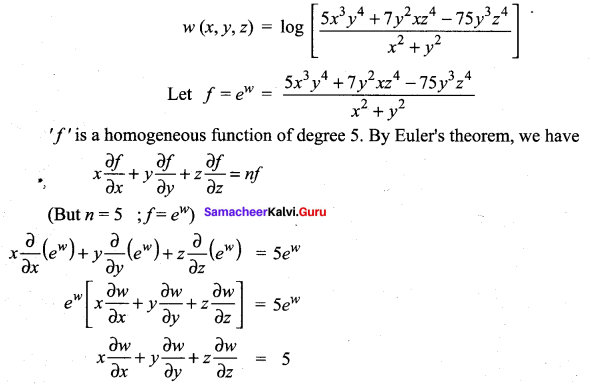### Samacheer Kalvi 12th Maths Solutions Chapter 8 Differentials and Partial Derivatives Ex 8.7 Additional Problems

Question 1.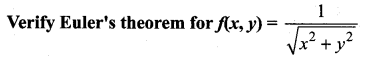Solution: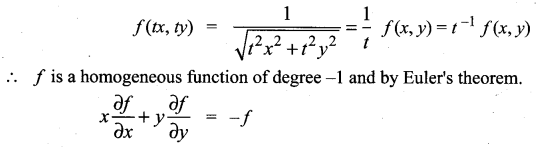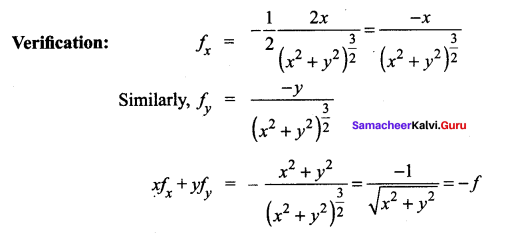Question 2.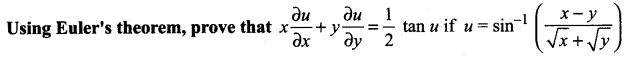Solution:
R.H.S. is not a homogeneous and hence